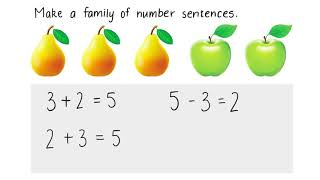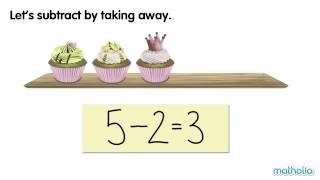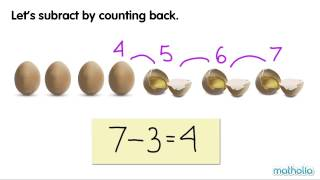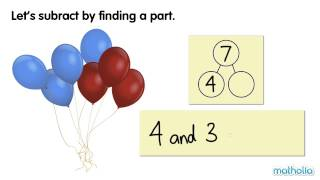## Practice and Learn

 1Number Sentences Video 2Subtract by Taking Away Video 3Subtracting by Taking Away Sheet 4Subtract by Counting Back Video 5Subtracting by Counting Back Sheet 6Subtract by Finding a Part Video 7Subtraction Equations Sheet 8Vertical Subtraction Sheet 9Subtraction Word Problems Sheet